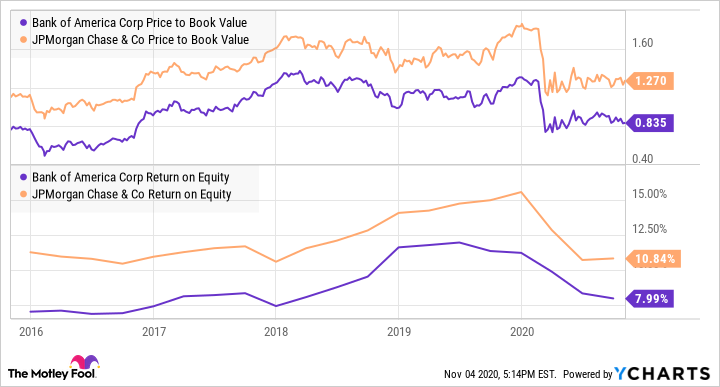You can calculate the price-to-book, or P/B, ratio by dividing a company's stock price by its book value per share, which is defined as its total assets minus any liabilities. This can be useful when you're conducting a thorough analysis of a stock.

## Why use the price-to-book ratio?

In a nutshell, a lower price-to-book ratio could indicate that a stock is undervalued. When you're comparing two stocks with similar growth and profitability, P/B can be useful for determining which is the best value at a given moment.Image source: Getty Images.

The lower a company's price-to-book ratio is, the better a value it generally is. This can be especially true if a stock's book value is less than one, meaning that it trades for less than the value of its assets. Buying a company's stock for less than book value can create a "margin of safety" for value investors. However, a very low P/B ratio can also be a sign of trouble at a company, so it should be used as part of a thorough stock analysis.

Like the price-to-sales ratio, the price-to-book ratio can be a particularly useful metric for a company with inconsistent or negative earnings; other common metrics like the price-to-earnings ratio aren't as meaningful in these situations. For example, many bank stocks have extremely inconsistent earnings, so the P/B ratio can give a clearer picture of the relative value of these companies.

## How to calculate price-to-book value

Book value is equal to a company's current market value divided by the "book value" of all of its shares. To determine a company's book value, you'll need to look at its balance sheet. Also known as shareholder's equity or stockholder's equity, this amount is equal to the company's assets minus its liabilities.Next, divide the book value by the number of outstanding shares in order to find the company's book value on a per-share basis so you can compare it with the current share price.Finally, divide the company's current stock price by the book value per share.### Example

Let's calculate the P/B ratio for Company X, which has:

• Total assets of \$3 billion
• Total liabilities of \$2 billion
• 100 million outstanding shares
• A current share price of \$15

We start by calculating Company X's book value, by subtracting \$2 billion (liabilities) from \$3 billion (assets) to get a book value of \$1 billion. Dividing that \$1 billion by the 100 million outstanding shares gives us a per-share book value of \$10. Finally, we divide the current share price of \$15 by that \$10 to reach a price-to-book multiple of 1.5.

## A word of caution

Price to book is useful only for evaluating certain types of businesses. If most of a business's assets are intangible -- as is the case with many technology companies -- its price to book may be unhelpfully high. Software giant Microsoft, for example, trades for more than 10 times its book value. On the other hand, price to book can be useful for capital-intensive businesses like banks.

A P/B ratio analysis doesn't tell us much all by itself. To get a more complete picture of a company's valuation, you should use it in combination with profitability metrics such as return on equity (ROE). For example, for the last five years, Bank of America's price-to-book multiple has been lower than JP Morgan Chase's. However, that doesn't necessarily mean that Bank of America is "cheaper." In fact, JP Morgan's ROE has been consistently higher than Bank of America's.While price to book can be a useful metric to have in your toolbox, it's only part of the puzzle when it comes to evaluating which stocks are undervalued.